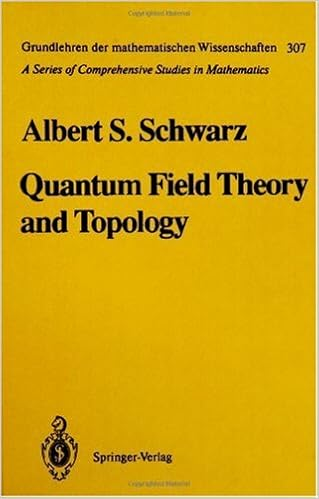## Wednesday, September 23, 2015

### Quantum Field Theory and Topology (Grundlehren der mathematischen Wissenschaften) by Albert S. Schwarz , E. Yankowsky , S. LevyIn recent years topology has firmly established itself as an important part of the physicist's mathematical arsenal. It has many applications, first of all in quantum field theory, but increasingly also in other areas of physics. The main focus of this book is on the results of quantum field theory that are obtained by topological methods. Some aspects of the theory of condensed matter are also discussed. Part I is an introduction to quantum field theory: it discusses the basic Lagrangians used in the theory of elementary particles. Part II is devoted to the applications of topology to quantum field theory. Part III covers the necessary mathematical background in summary form. The book is aimed at physicists interested in applications of topology to physics and at mathematicians wishing to familiarize themselves with quantum field theory and the mathematical methods used in this field. It is accessible to graduate students in physics and mathematics.